How to perform data validation using python by sorting columns on name?
BIG DATA RECIPES DATA CLEANING PYTHON DATA MUNGING MACHINE LEARNING RECIPES PANDAS CHEATSHEET     ALL TAGS

# How to perform data validation using python by sorting columns on name?

This recipe helps you perform data validation using python by sorting columns on name

## Recipe Objective

In most of the big data scenarios , Sorting/ordering is a process of arranging items systematically, and has two common, yet distinct meanings: ordering: arranging items in a sequence ordered by some criterion; categorizing: grouping items with similar properties. Ordering of the field names is extremely required before storing the data in the required order , so that it can be further processed by creating schema on top of it and doing further processing.

## System requirements :

• Install the python module as follows if the below modules are not found:
• `pip install pandas`
• The below codes can be run in Jupyter notebook, or any python console

## Step 1: Import the module

In this scenario we are going to use pandas numpy and random libraries import the libraries as below :

``` import pandas as pd ```

## Step 2 :Prepare the dataset

Data of Output looks as follows: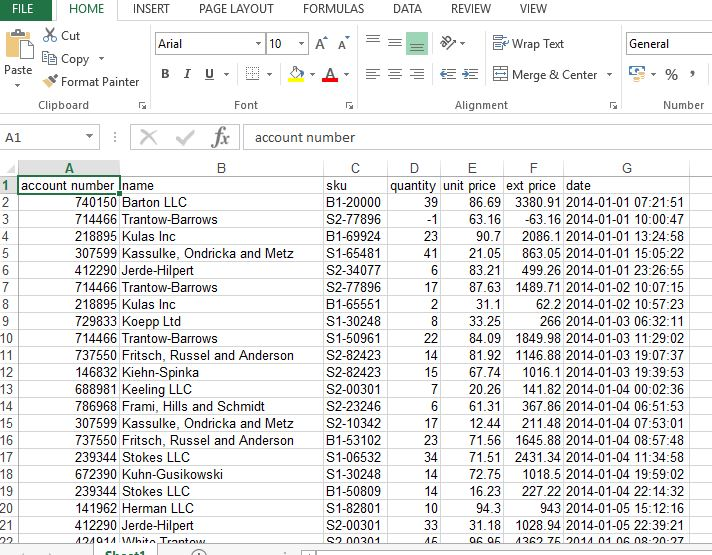Reading the excel file using pandas library as below and storing data as data frame and print 5 line of data.

``` df = pd.read_excel('sales_data.xlsx') df.head() ```

Output of the above code :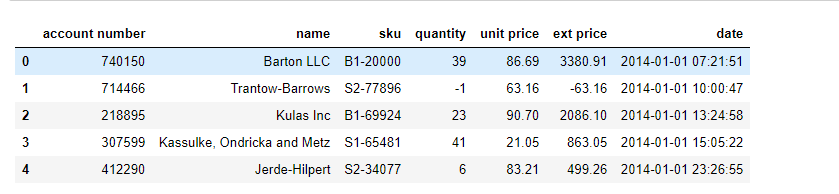To print only the column names type df.columns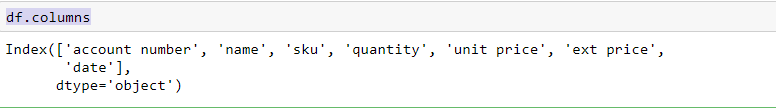Here in the code below we are going to add underscore instead of space using the replace method and to convert the column names into uppercase upper method.

``` for col in df.columns: df.rename(columns={col:col.upper().replace(" ","_")},inplace=True) print(df.columns) ```

Output of the above code :## Step 4: Arrange or sorting the columns

Here we are going to sort and arrange the columns based on the column name, so it means that we arrange the columns in alphabetical order.

``` df = df[sorted(df)] validation = df validation['chk'] = validation['ACCOUNT_NUMBER'].apply(lambda x: True if x in df else False) validation = validation[validation['chk'] == True].reset_index() df ```

Output of the above code :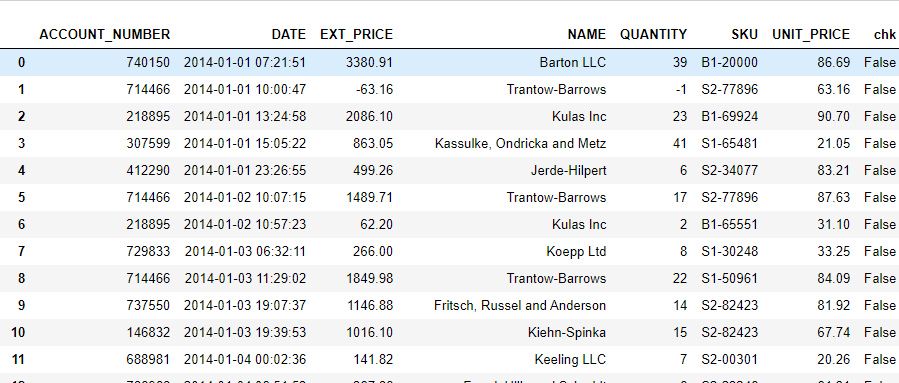Create a new dataframe and rename the column names as below

``` renamed_data = df.rename(columns = {'ACCOUNT_NUMBER': 'acnt_num','DATE':'buy_date','EXT_PRICE':'ext_price','NAME':'acc_holder_name', 'QUANTITY':'quantity','SKU':'stock_keep_unit', 'UNIT_PRICE':'unit_price'}) renamed_data.columns renamed_data ```

Output of the above code :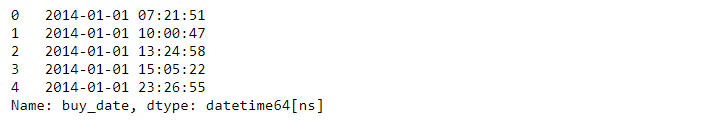## Step 5: Convert string to Date Data type and Select the row between dates

Here we have a column buy_date is string data type so we are going to convert the string data type date data type and we will select the row in between dates as follows below

Convert the string to Date datatype as below code:

``` renamed_data['buy_date'] = pd.to_datetime(renamed_data['buy_date']) renamed_data['buy_date'].head() ```

Output of the above code :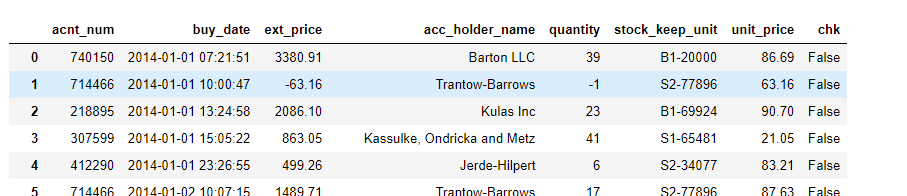Convert the string to Date datatype as below code:

``` start_date = pd.to_datetime('2014-01-08 17:46:40') end_date = pd.to_datetime('2014-01-23 20:40:21') renamed_data.loc[(renamed_data['buy_date'] > start_date) & (renamed_data['buy_date'] < end_date)] ```

Output of the above code :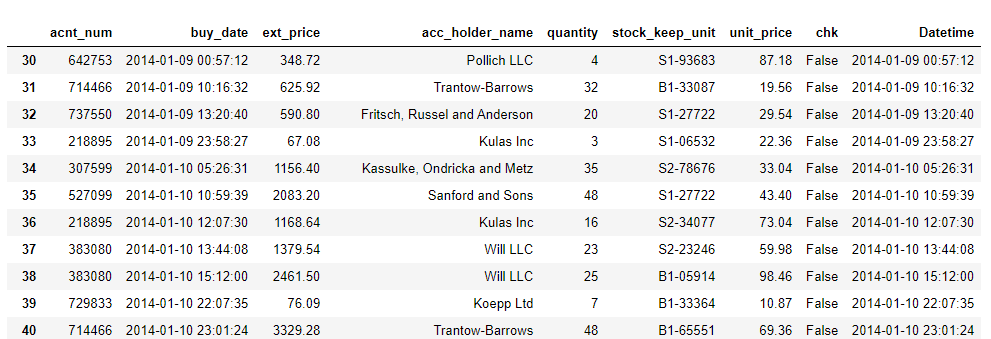#### Relevant Projects

In this hadoop project, we are going to be continuing the series on data engineering by discussing and implementing various ways to solve the hadoop small file problem.

##### Data processing with Spark SQL
In this Apache Spark SQL project, we will go through provisioning data for retrieval using Spark SQL.

##### Yelp Data Processing Using Spark And Hive Part 1
In this big data project, we will continue from a previous hive project "Data engineering on Yelp Datasets using Hadoop tools" and do the entire data processing using spark.

##### Tough engineering choices with large datasets in Hive Part - 2
This is in continuation of the previous Hive project "Tough engineering choices with large datasets in Hive Part - 1", where we will work on processing big data sets using Hive.

##### Building Real-Time AWS Log Analytics Solution
In this AWS Project, you will build an end-to-end log analytics solution to collect, ingest and process data. The processed data can be analysed to monitor the health of production systems on AWS.

##### Movielens dataset analysis for movie recommendations using Spark in Azure
In this Databricks Azure tutorial project, you will use Spark Sql to analyse the movielens dataset to provide movie recommendations. As part of this you will deploy Azure data factory, data pipelines and visualise the analysis.

##### Spark Project -Real-time data collection and Spark Streaming Aggregation
In this big data project, we will embark on real-time data collection and aggregation from a simulated real-time system using Spark Streaming.

##### Event Data Analysis using AWS ELK Stack
This Elasticsearch example deploys the AWS ELK stack to analyse streaming event data. Tools used include Nifi, PySpark, Elasticsearch, Logstash and Kibana for visualisation.

##### Real-time Auto Tracking with Spark-Redis
Spark Project - Discuss real-time monitoring of taxis in a city. The real-time data streaming will be simulated using Flume. The ingestion will be done using Spark Streaming.

##### Implementing Slow Changing Dimensions in a Data Warehouse using Hive and Spark
Hive Project- Understand the various types of SCDs and implement these slowly changing dimesnsion in Hadoop Hive and Spark.